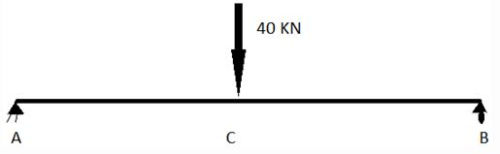Courses

# Test: Moment Area Theorems - 2

## 12 Questions MCQ Test | Test: Moment Area Theorems - 2

Description
This mock test of Test: Moment Area Theorems - 2 for Civil Engineering (CE) helps you for every Civil Engineering (CE) entrance exam. This contains 12 Multiple Choice Questions for Civil Engineering (CE) Test: Moment Area Theorems - 2 (mcq) to study with solutions a complete question bank. The solved questions answers in this Test: Moment Area Theorems - 2 quiz give you a good mix of easy questions and tough questions. Civil Engineering (CE) students definitely take this Test: Moment Area Theorems - 2 exercise for a better result in the exam. You can find other Test: Moment Area Theorems - 2 extra questions, long questions & short questions for Civil Engineering (CE) on EduRev as well by searching above.
QUESTION: 1

Solution:

QUESTION: 2

### Point A is pin joint and B is roller. A load of 40KN is applied at point C. Length of AC is 2m and d same goes for beam CB.Q.  What is the shape of BMD for this diagram?

Solution:

Explanation: It will increase till point C and then will start decreasing and in both cases linearly.

QUESTION: 3

### Point A is pin joint and B is roller. A load of 40KN is applied at point C. Length of AC is 2m and d same goes for beam CB.Q.   Where is the peak point of SFD?

Solution:

Explanation: Value of shear force at point A is +20KN which is the maximum for this case.

QUESTION: 4

Point A is pin joint and B is roller. A load of 40KN is applied at point C.
Length of AC is 2m and d same goes for beam CB.Q.   What is the peak value of BMD of this figure (all options are in KN-m)?

Solution:

Explanation: At point A BM will be 0 and hen will start increasing till point C where its value will be 40/EI i.e. the area between SFD till that point.

QUESTION: 5

Point A is pin joint and B is roller. A load of 40KN is applied at point C.
Length of AC is 2m and d same goes for beam CB.Q.  What should be the area that should be considered if we want to find slope at point B wrt initial beam?

Solution:

Explanation: If we thee deflected diagram of this figure, we will find that slope at point C will be zero as from that point, BM starts decreasing. So we should consider area between point C and B for slope wrt initial beam.

QUESTION: 6

Point A is pin joint and B is roller. A load of 40KN is applied at point C.
Length of AC is 2m and d same goes for beam CB.Q. What is the value of slope at point B wrt initial beam?

Solution:

Explanation: Just calculate the area between point C and point B.

QUESTION: 7

Point A is pin joint and B is roller. A load of 40KN is applied at point C.
Length of AC is 2m and d same goes for beam CB.Q.  To calculate maximum deformation in the deflected beam, which part of the area should be considered?

Solution:

Explanation: Maximum deformation happens at point C and slope of C is zero i.e. tangent is parallel to initial beam. This property of point C can be used to calculate maximum deformation.

QUESTION: 8

Point A is pin joint and B is roller. A load of 40KN is applied at point C.
Length of AC is 2m and d same goes for beam CB.Q.  To calculate maximum deformation in deflected beam, about which point should we take moment of the required part of area?

Solution:

Explanation: Tangent at point A is at point A as there is no vertical deflection there and tangent of point C is parallel to initial beam. So, the length at which it will cut point A vertically will be the maximum deformation.

QUESTION: 9

Point A is pin joint and B is roller. A load of 40KN is applied at point C.
Length of AC is 2m and d same goes for beam CB.Q. What is the value of maximum deformation in this case?

Solution:

Explanation: Just take area between point A and C and calculate moment about point A.

QUESTION: 10

Point A is pin joint and B is roller. A load of 40KN is applied at point C.
Length of AC is 2m and d same goes for beam CB.Q. To calculate relative deflections of tangents at point A and B at point B, about which point should we moment of the required part of area?

Solution:

Explanation: This is the basic second theorem of moment-area theorem.

QUESTION: 11

Point A is pin joint and B is roller. A load of 40KN is applied at point C.
Length of AC is 2m and d same goes for beam CB.Q. To calculate relative deflections of tangents at point A and B at point B, which part of area should be considered?

Solution:

Explanation: This is the basic first theorem of the moment-area theorem.

QUESTION: 12

Point A is pin joint and B is roller. A load of 40KN is applied at point C.
Length of AC is 2m and d same goes for beam CB.Q.  What will be the value of relative deflections of tangents at point A and B at point B?

Solution: